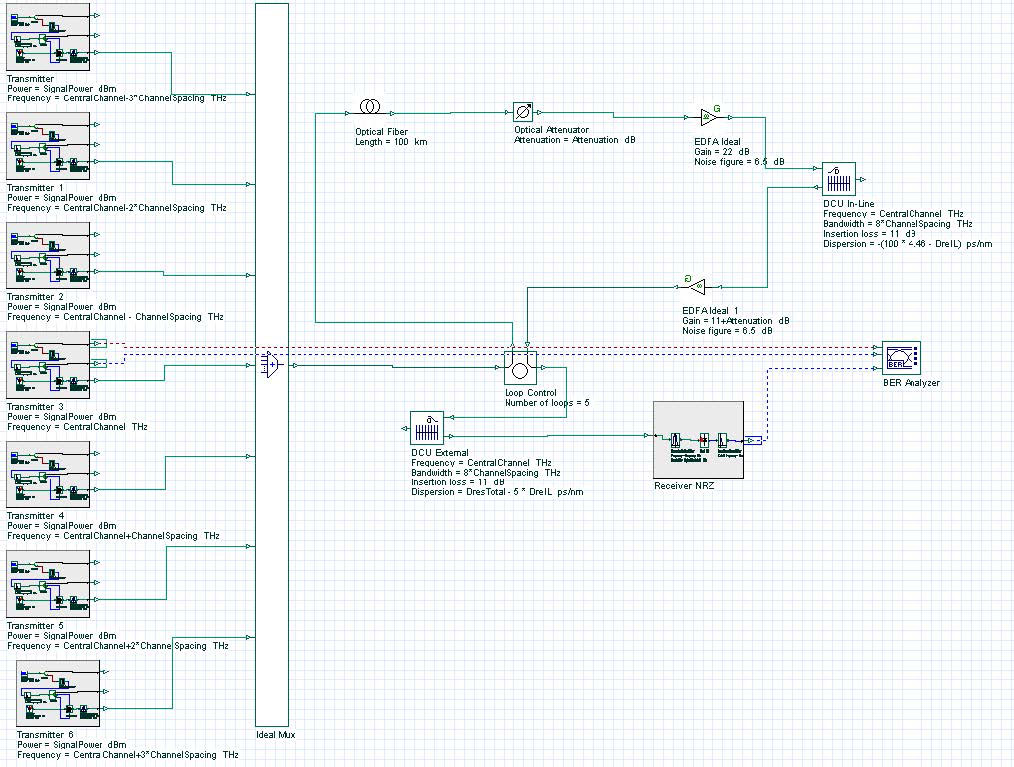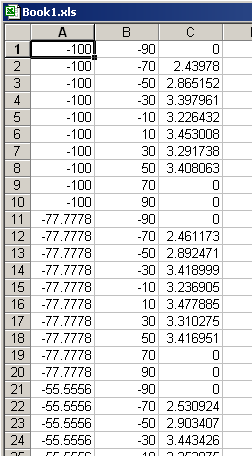The objective of this lesson is to demonstrate the performance analysis of a system using the script page to vary the system parameters and store the results.

Sometimes, to analyze the system performance, several parameters in the system are varied and the results for each different configuration have to be stored.

One way to perform these simulations is to put the parameters in sweep mode.

This method can be very effective if we have few parameters to analyze, but when the number of parameters increases, this kind of simulation can become too complex to setup all possible combination of values for each parameter.

Another issue is that all results are stored during the simulations, which can cause the simulations to slowdown because of the increase in the memory used.

The other way to do the simulation is to use the script page to set up the parameter values and store only the necessary results. In this method, the parameter setup is easier and you can specify which results you want to be stored.

In this example, we have 7 channels being propagated along 5 spans with 100 km of fiber and 2 dispersion compensation units (DCU). See Figure 1.Figure 1: System layout

In this example, we want to vary the dispersion value for each DCU. To do that, we must programme in the script page to vary the parameters “DreIL” and “DresTotal”. These are the dispersion of the DCU in line and the DCU external, respectively.

In the code, we select to save the result “Max. Q Factor” from the BER Analyzer and the way the parameters will be varied.

Finally, we allow the parameters and results to be stored in the spreadsheet software

Microsoft Excel. The following code was written in the script page.

‘OptiSystem Script: Nested Cloops with 2 parameters and 1 result
‘Parameters and Results can be exported to Excel or as Text
‘Global parameters – Parameter and Result names

ComponentName1 = “Layout 1”
ParameterName1 = “DreIL” ParameterName2 = “DresTotal”
VisualizerName1 = “BER Analyzer” ResultName1 = “Max. Q Factor”

‘Global parameters – Sweep ranges for parameters
NumberOfSweepIterations1 = 10
ParameterStart1 = -100
ParameterEnd1 = 100
NumberOfSweepIterations2 = 10
ParameterStart2 = -90
ParameterEnd2 = 90

‘Internal parameters – step for each sweep
ParameterStep1 = ( ParameterEnd1 – ParameterStart1 ) / ( NumberOfSweepIterations1 – 1 ) ParameterStep2 = ( ParameterEnd2 – ParameterStart2 ) / ( NumberOfSweepIterations2 – 1 )

‘Create Excel application – visible must be FALSE
Dim Excel
Set Excel = CreateObject(“Excel.Application”)
Excel.Visible = false

‘ OptiSystem SDK specifics – access components and visualizers
Dim LayoutMgr
Set LayoutMgr = Document.GetLayoutMgr
Dim Layout
Set Layout = LayoutMgr.GetCurrentLayout
Dim Canvas
Set Canvas = Layout.GetCurrentCanvas
Dim Component1
Set Component1 = Canvas.GetComponentByName(ComponentName1)
Dim Visualizer1
Set Visualizer1 = Canvas.GetComponentByName(VisualizerName1)

‘ Calculation loop – access parameters and results
Count = 1
For i = 0 to NumberOfSweepIterations1 – 1
For j = 0 to NumberOfSweepIterations2 – 1

‘Parameter Values
ParameterValue1 = ( ParameterStart1 + i * ParameterStep1 ) ParameterValue2 = ( ParameterStart2 + j * ParameterStep2 )

‘Set component parameters
Layout.SetParameterValue ParameterName1, ParameterValue1 * 1.0
Layout.SetParameterValue ParameterName2, ParameterValue2 * 1.0

‘Calculate
Document.CalculateProject TRUE , TRUE

‘Access visualizer results
Set Result1 = Visualizer1.GetResult( ResultName1 )

‘Access resut values
ResultValue1 = Result1.GetValue( 1 )

‘Send parameters and results to Excel
Excel.ActiveWorkbook.Worksheets(“sheet1”).Cells(Count,1) = ParameterValue1
Excel.ActiveWorkbook.Worksheets(“sheet1”).Cells(Count,2) = ParameterValue2
Excel.ActiveWorkbook.Worksheets(“sheet1”).Cells(Count,3) = ResultValue1
Count = Count + 1
Next
Next

‘Enable Excel application – visible must be TRUE
Excel.Visible = true

Part of the results obtained when running the script can be seen in Figure 2. In Figure

2, column A is the “DreIL”, column B is the “DresTotal” and column C is the Q factor.Figure 2: Parameters and results stored